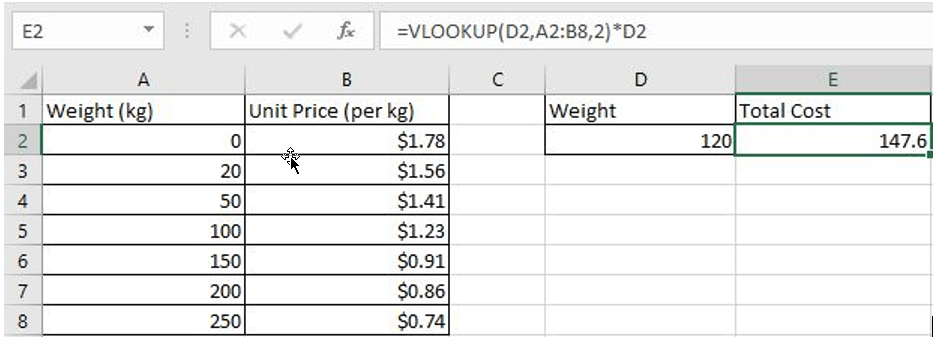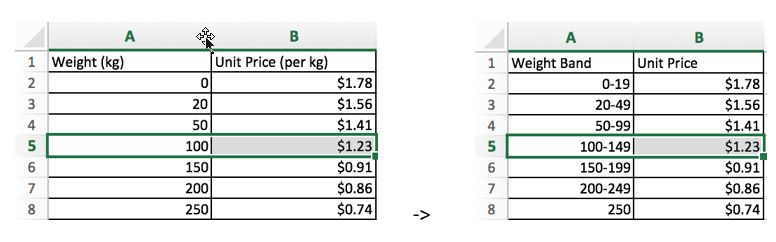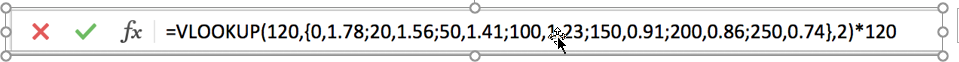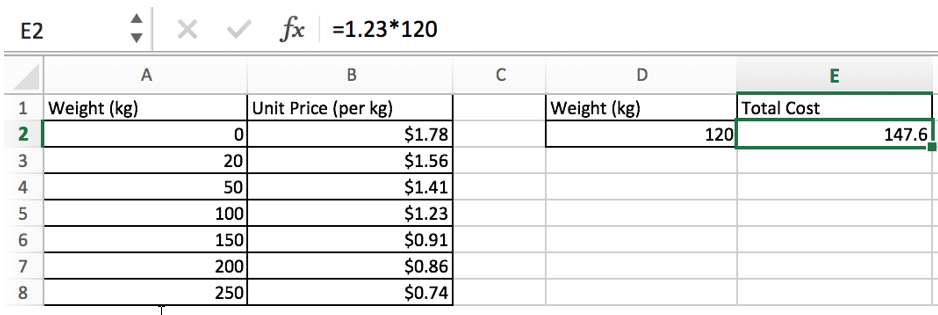# Calculate Total Cost with Excel VLOOKUP Function

In today’s article we will show you how to calculate the total cost for a given weight using the Excel VLOOKUP function. This function will help us to find the appropriate unit price for that weight and then we can use the simple multiplication “unit price * weight” to get the total cost.

Suppose we want to purchase a batch of something, unit prices are different depending on the weight bands, usually the unit price becomes cheaper as the weight increases.

If the correspondence between weight band and unit price is provided in a table in excel worksheet, how can we find the matching unit price and calculate the total price if we know the weight? Can we just create a simple formula to get the total cost?

Look at the example below. To calculate the total cost of something with a weight of 120kg.## FORMULA

In this example, the formula is:

`=VLOOKUP(D2,A2:B8,2)*D2`

### VLOOKUP INTRODUCTION

VLOOKUP function is a vertical lookup function in Excel, it runs a column-by-column lookup that eventually returns the value corresponding to the looked-up value in the specified column.

Syntax:

`=VLOOKUP(lookup_value,table_array,col_index_num,[range_lookup])`

Arguments:

• lookup_value is the value that needs to be looked up. Make sure it is in the first column of the table. lookup_value can be a numeric value, a reference, or a text string.
• table_array is the table in which the data needs to be looked up. Use a range reference to or a table name to represents the table.
• col_index_num is the column number of the data to be looked up in the table_array.
• range_lookup provides two modes for VLOOKUP; If range_lookup is TRUE or 1, the VLOOKUP function will look for an approximate match; if range_lookup is FALSE or 0, VLOOKUP returns an exact match; and it returns an error value #N/A if not found. If this argument is omitted, the default value is 1, which means that VLOOKUP returns an approximate match.

## EXPLANATION

As we mentioned above, the total cost is equal to the unit price * weight. In this example, the unit price is not a fixed value, it is determined by the weight, and since the weight is given by D2, we need to know the unit price of D2, and we use Excel VLOOKUP function to retrieve the corresponding unit price.

So, in this formula:

VLOOKUP(D2,A2:B8,2) – unit price

D2 – weight of the input

In VLOOKUP(D2,A2:B8,2)

• Lookup_value – D2 (120kg)
• Table_array – A2:B8
• col_index_num – 2 (returns the unit price as unit price in the second column)
• range_lookup – 1- Approximate match (If this argument is omitted, the default value is 1)

### Step#1 VLOOKUP finds the row of entered weight “120”

In this example, “120” is not listed in the first column of the table. Since the value of range_lookup is 1, VLOOKUP returns an approximate match. In approximate match mode, Excel VLOOKUP function will search for “120” in the first column until it encounters the first value greater than it, and then return to the previous row.

In this example, in “Weight” column, Excel VLOOKUP function compares each weight against with the lookup value “120”, and when coming to cell A6, it encounters the first value greater than “120” which is 150, so it returns to A5.(you can compare the two pictures for better understanding the approximate match)

### Step#2 VLOOKUP returns unit price in the row of weight “120”

In the previous step, we know that the row index of weight “120” is 5. Since the column index is 2, Excel VLOOKUP returns the intersection in row 5 and column 2 of table A2:B8. The intersection is B2, value in B2 is 1.23. So VLOOKUP function returns 1.23 finally.

### Step#3 Calculate the formula =VLOOKUP(D2,A2:B8,2)*D2The formula is equivalent to

`=VLOOKUP(120,{0,1.78;20,1.56;50,1.41;100,1.23;150,0.91;200,0.86;250,0.74},2)*120`In step#2, we know VLOOKUP returns 1.23, so the total cost in this example is 1.23*120=147.6.### Related Functions

• Excel VLOOKUP function
The Excel VLOOKUP function lookup a value in the first column of the table and return the value in the same row based on index_num position.The syntax of the VLOOKUP function is as below:= VLOOKUP (lookup_value, table_array, column_index_num,[range_lookup])….